# The R/2nR DAC: Binary-Weighted-Input Digital-to-Analog Converter

## Chapter 13 - Digital-Analog Conversion

### What Is a R/2nR DAC Circuit?

The R/2nR DAC circuit, otherwise known as the binary-weighted-input DAC, is a variation on the inverting summing op-amp circuit. (Note that "summing" circuits are sometimes also referred to as "summer" circuits.)

If you recall, the classic inverting summing circuit is an operational amplifier using negative feedback for controlled gain, with several voltage inputs and one voltage output. The output voltage is the inverted (opposite polarity) sum of all input voltages: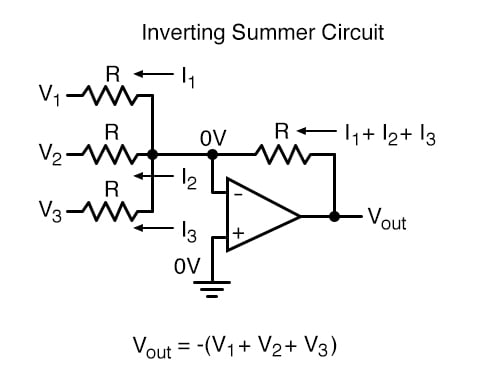For a simple inverting summing circuit, all resistors must be of equal value. If any of the input resistors were different, the input voltages would have different degrees of effect on the output, and the output voltage would not be a true sum.

### Example: An R/2nR DAC with Multiple Input Resistor Values

Let’s consider, however, intentionally setting the input resistors at different values. Suppose we were to set the input resistor values at multiple powers of two: R, 2R, and 4R, instead of all the same value R: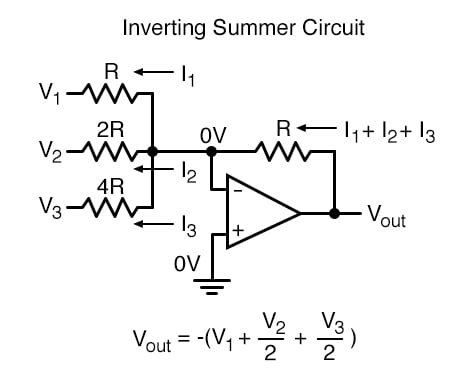Starting from V1 and going through V3, this would give each input voltage exactly half the effect on the output as the voltage before it. In other words, input voltage V1 has a 1:1 effect on the output voltage (gain of 1), while input voltage V2 has half that much effect on the output (a gain of 1/2), and V3 half of that (a gain of 1/4).

These ratios were not arbitrarily chosen: they are the same ratios corresponding to place weights in the binary numeration system.  If we drive the inputs of this circuit with digital gates so that each input is either 0 volts or full supply voltage, the output voltage will be an analog representation of the binary value of these three bits.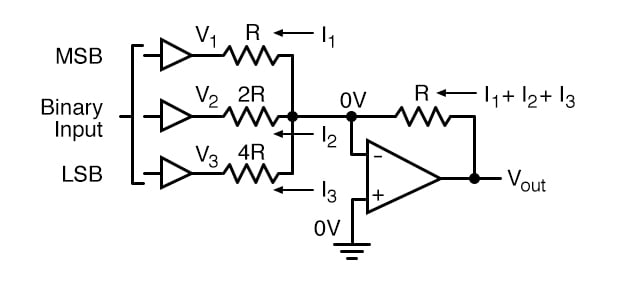If we chart the output voltages for all eight combinations of binary bits (000 through 111) input to this circuit, we will get the following progression of voltages:

-------------- | Binary | Output voltage | ----------- | 000 | 0.00 V  |
-------------- | 001    | -1.25 V | ------------------ | 010 | -2.50 V |
-------------- | 011    | -3.75 V | ------------------ | 100 | -5.00 V |
-------------- | 101    | -6.25 V | ------------------ | 110 | -7.50 V |
-------------- | 111    | -8.75 V | ------------------


Note that with each step in the binary count sequence, there results a 1.25 volt change in the output.

This circuit is very easy to simulate using SPICE. In the following simulation, I set up the DAC circuit with a binary input of 110 (note the first node numbers for resistors R1, R2, and R3: a node number of “1” connects it to the positive side of a 5 volt battery, and a node number of “0” connects it to ground).

The output voltage appears on node 6 in the simulation: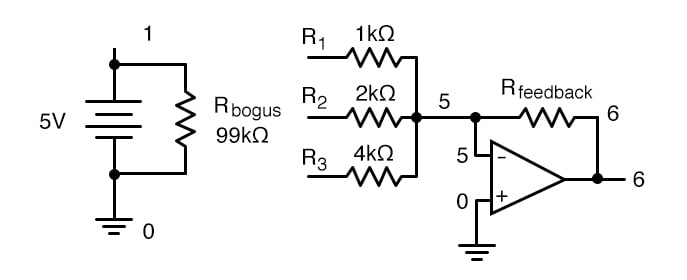binary-weighted dac v1 1 0 dc 5 rbogus 1 0 99k r1 1 5 1k r2 1 5 2k r3 0 5 4k rfeedbk 5 6 1k e1 6 0 5 0 999k .end node voltage node voltage node voltage (1) 5.0000 (5) 0.0000 (6) -7.5000

We can adjust resistors values in this circuit to obtain output voltages directly corresponding to the binary input. For example, by making the feedback resistor 800 Ω instead of 1 kΩ, the DAC will output -1 volt for the binary input 001, -4 volts for the binary input 100, -7 volts for the binary input 111, and so on.

(with feedback resistor set at 800 ohms) ------ | Binary | Output voltage |
---------------- | 000 | 0.00 V  | ------------ | 001    | -1.00 V |
---------------- | 010 | -2.00 V | ------------ | 011    | -3.00 V |
---------------- | 100 | -4.00 V | ------------ | 101    | -5.00 V |
---------------- | 110 | -6.00 V | ------------ | 111    | -7.00 V |
----------------


If we wish to expand the resolution of this DAC (add more bits to the input), all we need to do is add more input resistors, holding to the same power-of-two sequence of values: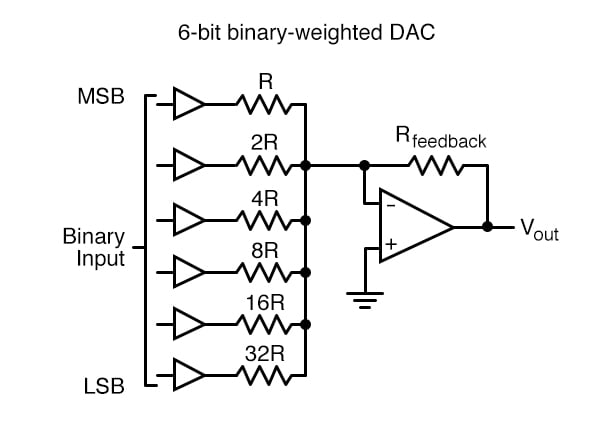It should be noted that all logic gates must output exactly the same voltages when in the “high” state.  If one gate is outputting +5.02 volts for a “high” while another is outputting only +4.86 volts, the analog output of the DAC will be adversely affected.

Likewise, all “low” voltage levels should be identical between gates, ideally 0.00 volts exactly. It is recommended that CMOS output gates are used, and that input/feedback resistor values are chosen so as to minimize the amount of current each gate has to source or sink.

• Share
Published under the terms and conditions of the Design Science License